# The FEAST algorithm for Hermitian eigenproblems

## Introduction

FEAST is an algorithm for computing a few eigenpairs $(\lambda, x)$ of a large sparse eigenvalue problem $A x = \lambda B x$, where $A$ is a Hermitian $N\times N$ matrix, and $B$ is Hermitian positive definite . FEAST belongs to the class of contour-based eigensolvers which have recently attracted a lot of attention .

Mathematically, FEAST can be interpreted as a subspace iteration applied to a rational matrix function which serves the purpose of separating the wanted eigenvalues from the unwanted (i.e., it acts as a filter function). The RKFUN calculus of the RKToolbox is a convenient tool for working with rational functions and it allows for a simple implementation of the basic FEAST iteration. Of course, the full FEAST implementation comes with many more "bells and whistles," and in this tutorial we only aim to demonstrate the basic idea.

## Model eigenvalue problem

Let us consider an eigenvalue problem $A x =\lambda x$, where $A$ is a finite-difference discretization of the Laplacian on a disk with homogeneous Dirichlet boundary conditions. Using MATLAB's eigs function, we first compute and visualize the $9$ eigenvectors corresponding to the smallest eigenvalues of $A$:

n = 150;
G = numgrid('D', n);
A = (n+1)^2*delsq(G);
N = size(A, 1);
B = speye(N);
[V, D]   = eigs(A, 9, 'SM');
[D, ind] = sort(diag(D)); V = V(:, ind);
figure(1)
for j = 1:9
subplot(3, 3, j)
v = V(:, j);
U = NaN*G;
U(G>0) = full(v(G(G>0)));
contourf(U);
prism, axis square ij, axis off
title(['\lambda = ' num2str(D(j))]);
end## Constructing the filter function

Suppose we want to find these $9$ eigenmodes using FEAST, then we need to contruct a rational filter which would separate the corresponding eigenvalues of $A$ from the others. To this end, we combine a type $(9,10)$ step function approximation for the interval $[-1,1]$ with a linear transformation $t$ mapping $[0,220]$ to $[-1,1].$ The result is a near-optimal rational filter $r$ for the interval $[0,220]$, attaining values very close to $1$ on that interval, and oscillating about $0$ outside:

lmin = 0; lmax = 220;
x = rkfun();                                   % independent variable x
t = 2/(lmax-lmin)*x - (lmin+lmax)/(lmax-lmin); % map [lmin,lmax] -> [-1,1]
s = rkfun('step', 5);
r = s(t);
figure, ezplot(r), hold on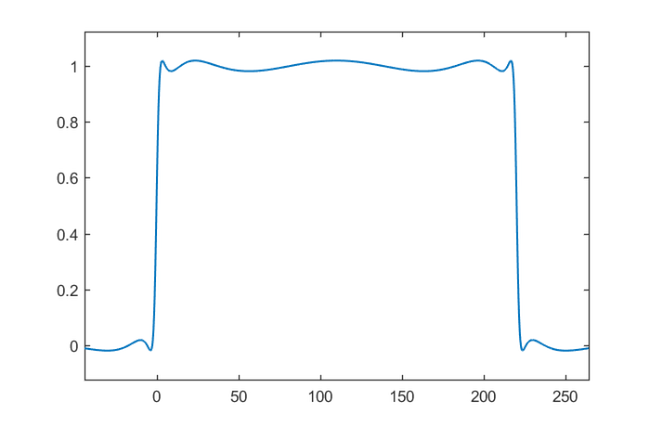Let's compute the separation ratio between the approximate values for $1$ and $0$ by finding the real local extrema of $r$, and then finding the smallest/largest modulus of $r$ inside/outside $[0,220]$ at these extrema. Such computations can become quite sensitive, in particular with rational functions of high degree. We therefore recommend performing this using the Advanpix Multipleprecision Toolbox :

r = mp(r);
extrema = roots(diff(r), 'real');
values  = r(extrema);
plot(extrema, values, 'ro')
mini = min(abs(r(extrema(lmin < extrema & extrema < lmax))));
maxi = max(abs(r(extrema(lmin > extrema | extrema > lmax))));
plot([lmin, lmax], [mini, mini], 'k--')
plot([-1e6, lmin], [maxi, maxi], 'k--')
plot([lmax,  1e6], [maxi, maxi], 'k--')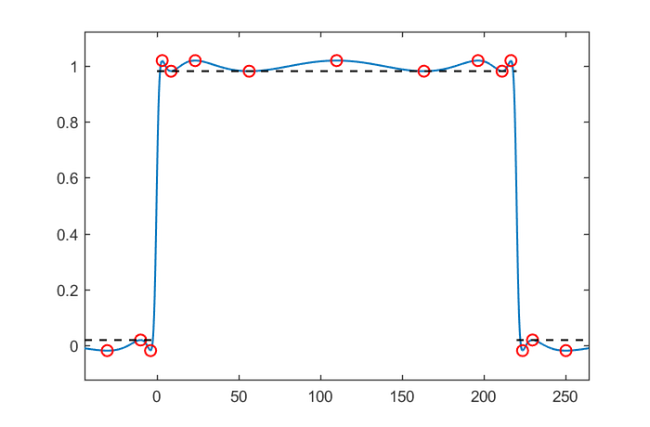According to , the approximate convergence factor of FEAST for finding the eigenpairs in the interior of $[0,220]$ is given by the following ratio:

ratio = double(maxi/mini)

ratio =
1.9351e-02


## A simple FEAST implementation

We can easily verify this numerically by implementing a simple subspace iteration applied to $r(A)$. Conveniently, the RKToolbox allows us to simply type $r(A,V)$ for computing $r(A)V$, the action of the rational filter on a basis $V$ for the search space.

% Change to double precision.
r = double(r);
% Search space basis V of dimension m.
m = 10;
V = randn(N, m);

for iter = 1:8
% Apply rational filter to V.
V = r(A, V);
% Compute and sort Ritz pairs.
Am = V'*A*V; Bm = V'*B*V;
[W, D]   = eig(Am, Bm);
[D, ind] = sort(diag(D)); W = W(:, ind);
% B-normalize W.
nrm = sqrt(diag(W'*Bm*W)); W = W/diag(nrm);
% Form approximate eigenvectors.
V = V*W;
% Check residuals and number of eigenpairs inside
% search iterval.
Di = diag(D(lmin < D & D < lmax));
Vi = V(:, lmin < D & D < lmax);
resid(iter) = norm(A*Vi - B*Vi*Di, 'fro');
nrvec(iter) = size(Vi, 2);
end


Here is a plot of the residual norms after each FEAST iteration, together with the expected convergence factor, and the number of Ritz values inside the search iterval:

figure
semilogy(resid, 'b-o'), hold on
semilogy(1e10*ratio.^(1:iter), 'k--')
for j = 1:iter
hdl = text(j-.1,5*resid(j), num2str(nrvec(j)));
set(hdl, 'FontSize', 14, 'Color', 'b')
end
xlim([.5, iter+.5]), xlabel('iteration')
legend('residual norm','expected rate')
ylim([1e-8, 1e6])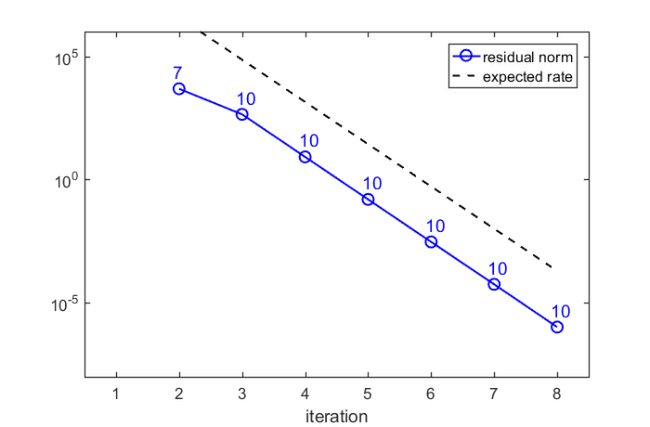There is a very good agreement between the predicted and observed convergence. In the first iteration, no Ritz value is in the search interval, but after only $3$ iterations we have found $10$ Ritz values. Indeed, looking at the 9th eigenvector in the plot above, we expect by symmetry that there will be a 10th eigenvalue corresponding to the same eigenvalue $\approx 202.3766$.

## Remarks on parallel implementation

As mentioned above, our basic FEAST implementation is not at all efficient and serves only the purpose of a demonstration. A main feature of FEAST is its parallelism in evaluating $r(A)V$ by using a partial fraction expansion of $r$ of the form

$\displaystyle r(z) = \frac{\alpha_1}{z-\beta_1} + \cdots + \frac{\alpha_n}{z-\beta_n}.$

We can easily compute such an expansion using the residue command of the RKToolbox, again in multiple precision to avoid accuracy loss. Here is a plot of the poles of $r$ in the complex plane, relative to the search interval $[0,220]$:

[alpha,beta] = residue(mp(r));
figure
plot(beta, 'ro'), hold on
plot([lmin, lmax], [0, 0], 'k-')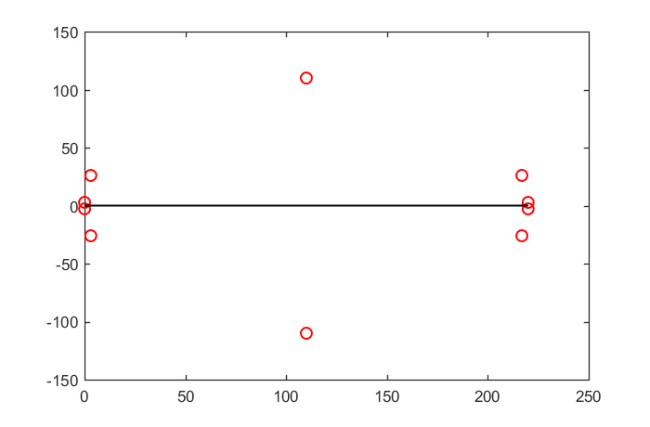That's it. The following command creates a thumbnail

figure(1), hold on, plot(NaN)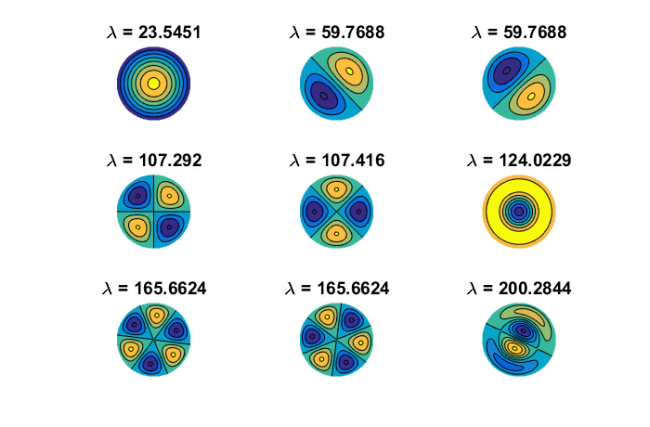## References

 Advanpix LLC., Multiprecision Computing Toolbox for MATLAB, ver 4.3.3.12213, Tokyo, Japan, 2017. http://www.advanpix.com/.

 S. Güttel, E. Polizzi, P. Tang, and G. Viaud. Zolotarev quadrature rules and load balancing for the FEAST eigensolver, SIAM J. Sci. Comput., 37(4):A2100--A2122, 2015.

 A. Imakura, L. Du, and T. Sakurai. A map of contour integral-based eigensolvers for solving generalized eigenvalue problems, http://arxiv.org/abs/1510.02572, 2015

 E. Polizzi. Density-matrix-based algorithm for solving eigenvalue problems, Phys. Rev. B, 79:115112, 2009.